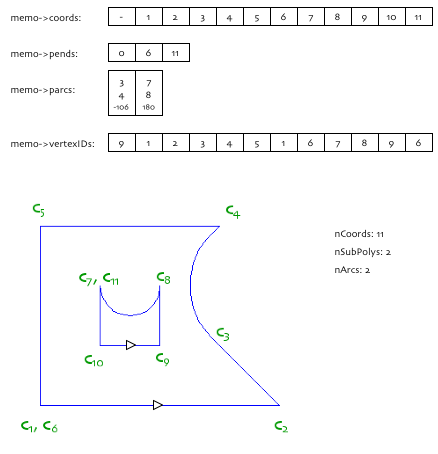## API_​Polygon

Representation of a two dimensional polygon.

```    typedef struct {
Int32          nCoords;
Int32          nSubPolys;
Int32          nArcs;
GS::IntPtr     filler_1;
} API_Polygon;
```

#### Members

nCoords
Number of elements in the coordinate array. The begin points of contours are duplicated.
nSubPolys
Number of closed subpolygons including the main polygon. For polylines, this is always 1.
nArcs
Number of arched segments in the polygon/polyline.

#### Remarks

This structure holds the size of the corresponding geometry only, i.e. the number of nodes, number of subpolygons and number of arc segments in the polygon shape. The geometry data is always passed through an API_ElementMemo structure.

What are the components:

• 2D coordinates; represented by an API_Coord array.
• subpolygons; represented by an index (Int32) array to the coordinates. Each index points to the last coordinate of a contour.
• arc segments; represented by an API_PolyArc array.

Let’s see an example, with the list of rules.Coordinates:

• `poly->nCoords = BMGetHandleSize ((GSHandle) memo->coords) / sizeof (API_Coord) - 1;`
• the first coordinate of the array is not used; must be initialized to (0.0, 0.0) for polygons, and to (-1.0, 0.0) for polylines,
• coordinates are duplicated for the end-nodes for each subcontour (see c1 and c7)

Contour ends:

• `poly->nSubPolys = BMGetHandleSize ((GSHandle) memo->pends) / sizeof (Int32) - 1;`
• the `pends` array contains indices to the coordinate array, indicating the vertices which close the subcontours
• the first element is always 0

Arc segments:

• `poly->nArcs = BMGetHandleSize ((GSHandle) memo->parcs) / sizeof (API_PolyArc);`
• the `parcs` array is allocated only if there are any arc segments in the polygon,
• each record refers to two coordinate indices (`begIndex`, `endIndex`) between which the polygon edge is an arc,
• each record gives the signed arc angle. The angle is positive, if the arc is on the right-hand side of the (begPoint, endPoint) segment,
• the angle is given in radians (degrees are shown on the picture only for better readability)

Vertex IDs:

• the number of coordinates and the number of vertexIDs are equal; each coordinate has a vertexID. That’s why the `API_Polygon` structure does not contain an `nVertexID` field,
• vertexIDs can be called as node unique IDs (that’s why c1 and c6 have the same vertex ID),
• the maximal vertexID value is stored in the 0. cell,
• there isn’t any correspondence between the coordinate indices and the assigned vertexIDs; however they are often the same. (See that c6 has the ID 1.),
• upon editing the polygon shape the maximal vertexID can be not be decremented, and the nodes must keep the vertexID values
• vertex IDs are useful in dimensioning and editing

Editing the shape of a polygon covers many difficulties which must be handled. They are:

• vertex IDs must be maintained,
• arc references must be updated; partially,
• contour-end references must be updated; partially.

Use the provided functions to change the shape of a polygon. These functions are:

#### Examples

``````
Int32 FindArc (const API_PolyArc *parcs, Int32 nArcs, Int32 node)
{
Int32        i;

if (parcs == nullptr)
return (-1);
for (i = 0; i < nArcs; i++)
if (parcs [i].begIndex == node)
return (i);
return (-1);
}

void TrackPoly (const API_Polygon *poly, const API_ElementMemo *memo)
{
int         j, i, begInd, endInd;
API_Coord   begC, endC;

for (j = 1; j <= poly->nSubPolys; j++) {
begInd = (*memo->pends) [j-1] + 1;
endInd = (*memo->pends) [j];
for (i = begInd; i < endInd; i++) {
begC = (*memo->coords) [i];
endC = (*memo->coords) [i+1];
arcInd = FindArc (*memo->parcs, poly->nArcs, i);
}
}
}
``````

The example above traverses through the polygon nodes, based on the subcontour references.

#### Requirements

Version: API 2.1 or later